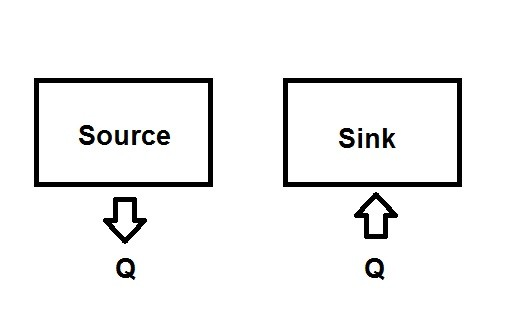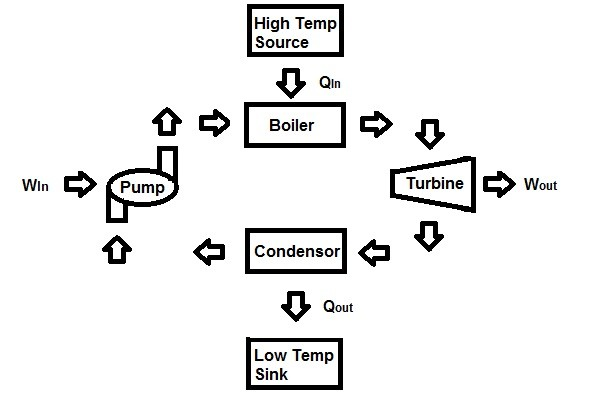# Second Law of Thermodynamics

Up to this point all of the topics have been based of the first law of thermodynamics (the conservation of energy). However, just because something follows the first law doesn’t mean that it can happen in nature. An example would be a hot cup of coffee left on your desk. Eventually that cup of coffee is going to cool down. However, by the conservation of energy equation then energy lost into the environment should be able to be placed back into that cup of coffee so that it reaches the temperature that it was originally at. Of course you know that this is impossible, but what I just said wouldn’t violate the first law. Instead to heat this cup of coffee again you need to either put it in an environment that is at a higher temperature, or you need convert work to heat. Both of these conditions cause a loss of energy in the form of heat that cannot be reversed. This means that real life processes have a certain direction that energy can flow, and that energy has certain quality or efficiency to the quantity of energy that is transferred. This is essentially what the second law of thermodynamics describes.

The Kelvin Planck Statement can be used as a more formal definition of the second law. “No heat engine can have a thermal efficiency of 100%, or for a power plant to operate, the working fluid must exchange heat with the environment as well as the furnace.”

##### Thermal Energy Reservoirs

When dealing with the second law of thermodynamics you must describe a thermal reservoir in your problem; one of each type. There are two types of thermal reservoirs; a source and a sink. A source is a thermal reservoir that provides thermal energy to your process, while a sink is a thermal reservoir that removes heat from your process.For something to be considered a thermal reservoir its properties must remain constant. Objects that are large such as a lake, the ocean, or the atmosphere can be considered thermal reservoirs due to their size. However, size does not dictate whether something is a thermal reservoir. For example, furnaces or boilers can also be considered thermal reservoirs a long as their properties are regulated.

Finally, as a side note, since rivers, lakes, and the ocean are common thermal sinks for plants that have waste energy thermal pollution can become an issue. Thermal pollution is caused by energy being dumped into the thermal sink to fast so that the energy cannot disperse fast enough causing the temperature to rise. The rising temperature can hurt or kill marine life, which means this must be considered when designing a thermodynamic process for a plant, so that it will comply with environmental laws.

##### Heat Engine

Work energy can easily be converted to heat and other forms of energy, but converting energy back to work is not an easy task. However, taking the second law of thermodynamics into consideration, it is possible to create what is called a heat engine to transform energy into work. For a heat engine to work its process has to be the following.

1. Receive heat from a high temperature source, such as a furnace or boiler.

2. Part of that heat will then be converted into work, such as steam turning a turbine shaft.

3. The remaining heat will have to rejected as waste energy into a sink, so that the process can repeat.

A working fluid, such as water would be used so that the heat can be transferred from the source, and the waste heat can transferred to the sink. Below a schematic of a heat engine can be seen.Qin = amount of heat supplied by furnace or boiler

Qout = amount of heat rejected from the system through the sink

Wout = Amount of work delivered by the steam as it expands through the turbine

Win = Work required to run the pump

##### Thermal Efficiency

The second law of thermodynamic states that Qout can never equal zero. This means that no process that converts energy into work can be 100% efficient. To calculate thermal efficiency the following equation would be used.

(Eq 1)   $η_{th}=\frac{W_{net, out}}{Q_{in}}=1-\frac{Q_{out}}{Q_{in}}$

In all real life systems some of the input energy is wasted, and is very difficult to save Qout since it must be reject to complete the cycle. For example, most auto engines have a 25% efficiency, while diesel engines have a 40% efficiency, and finally a well designed gas turbine power plant can have a 60% efficiency.